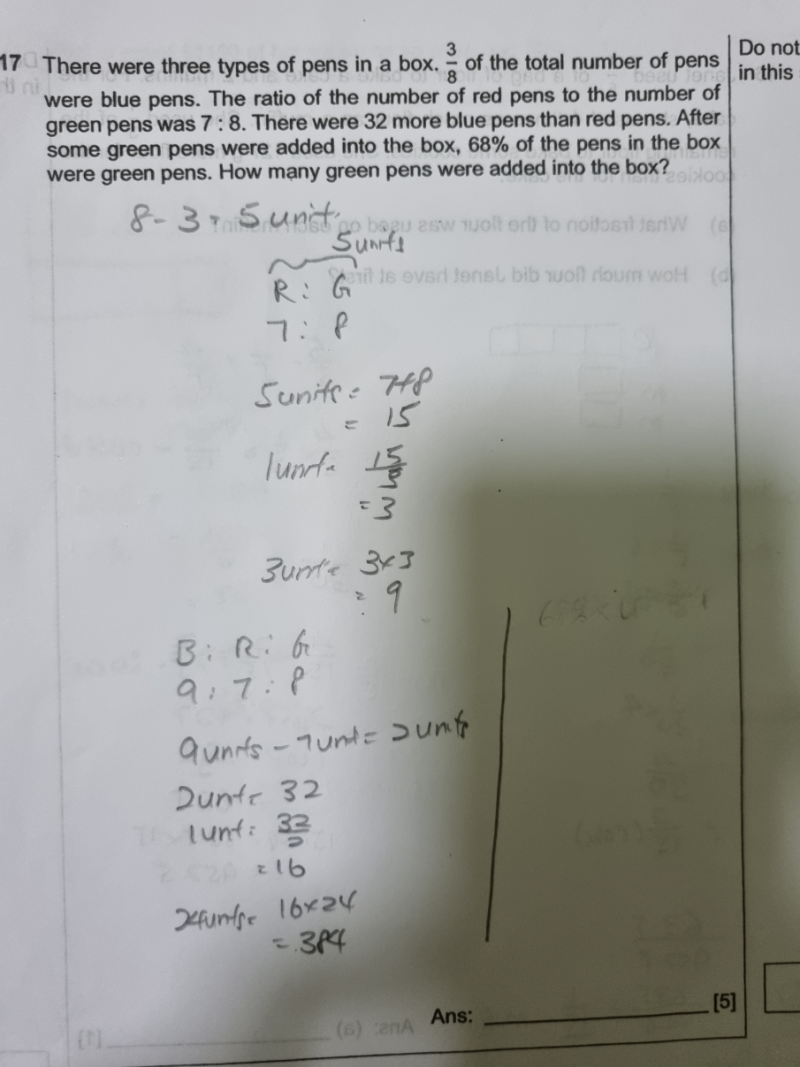# QuestionAre my first steps correct?the next I am confused .pls help thanks

The first part is correct but it can be shortened in working in a diagram form alternatively.

B               R          G

3            <–5units–>     (x 3 to make them equal = 15 units)

x3 =9u             7u          8u            (total = 24u)

<–15units->

So 9u-7u = 32 => u = 16

24u =384,  8u = 128

Alternative Solution for part 2:

Let p be the added green pens

(128+p)/(383+p) = 68/100

12800 + 100p = 26112 + 68p

32p =13312   => p =416

Hence number of green pens added is 416.

0 Replies 1 Like